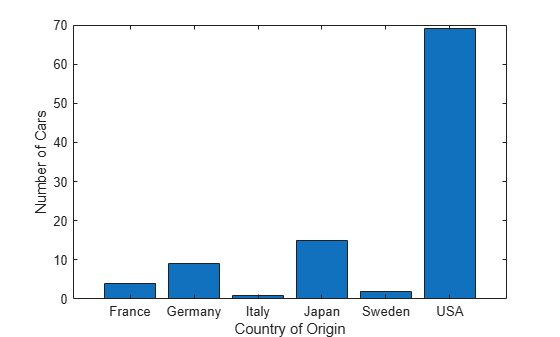# tabulate

Frequency table

## Syntax

``tabulate(x)``
``tbl = tabulate(x)``

## Description

example

````tabulate(x)` displays a frequency table of the data in the vector `x`. For each unique value in `x`, the `tabulate` function shows the number of instances and percentage of that value in `x`. See `tbl`.```

example

````tbl = tabulate(x)` returns the frequency table `tbl` as a numeric matrix when `x` is numeric and as a cell array otherwise.```

## Examples

collapse all

Create a frequency table for a vector of data.

Load the `patients` data set. Display the first five entries of the `Gender` variable. Each value indicates the gender of a patient.

```load patients Gender(1:5)```
```ans = 5x1 cell array {'Male' } {'Male' } {'Female'} {'Female'} {'Female'} ```

Generate a frequency table that shows the number and percentage of `Male` and `Female` patients in the data set.

`tabulate(Gender)`
``` Value Count Percent Male 47 47.00% Female 53 53.00% ```

Create a frequency table for a vector of positive integers. By default, if a vector `x` contains only positive integers, then `tabulate` returns 0 counts for the integers between `1` and `max(x)` that do not appear in `x`. To avoid this behavior, convert the vector `x` to a `categorical` vector before calling `tabulate`.

Load the `patients` data set. Display the first five entries of the `Height` variable. Each value indicates the height, in inches, of a patient.

```load patients Height(1:5)```
```ans = 5×1 71 69 64 67 64 ```

Create a frequency table that shows, in its second and third columns, the number and percentage of patients in the data set that have a particular height. Display the first five entries and the last five entries of the matrix that `tabulate` returns. `tbl` contains one row for each height between `1` and `72` inches, where `72` is the maximum height value in `Height`.

```tbl = tabulate(Height); first = tbl(1:5,:)```
```first = 5×3 1 0 0 2 0 0 3 0 0 4 0 0 5 0 0 ```
`last = tbl(end-4:end,:)`
```last = 5×3 68 15 15 69 8 8 70 11 11 71 10 10 72 4 4 ```

Generate a frequency table that shows `Count` and `Percent` values only for heights that appear in the `Height` variable. Convert `Height` to a `categorical` variable, and then call the `tabulate` function.

```newHeight = categorical(Height); tabulate(newHeight)```
``` Value Count Percent 60 1 1.00% 62 3 3.00% 63 7 7.00% 64 12 12.00% 65 8 8.00% 66 15 15.00% 67 6 6.00% 68 15 15.00% 69 8 8.00% 70 11 11.00% 71 10 10.00% 72 4 4.00% ```

Create a frequency table from a character array by using `tabulate`. Convert the resulting cell array to a `table` array, and visualize the results.

Load the `carsmall` data set. Tabulate the data in the `Origin` variable, which shows the country of origin of each car in the data set. Convert the resulting cell array `tbl` to a `table` array `t`. Change the `Value` column to a `categorical` vector.

```load carsmall tbl = tabulate(Origin); t = cell2table(tbl,'VariableNames', ... {'Value','Count','Percent'}); t.Value = categorical(t.Value)```
```t=6×3 table Value Count Percent _______ _____ _______ USA 69 69 France 4 4 Japan 15 15 Germany 9 9 Sweden 2 2 Italy 1 1 ```

Create a bar graph from the frequency table.

```bar(t.Value,t.Count) xlabel('Country of Origin') ylabel('Number of Cars')```Create a frequency table from a numeric vector with `NaN` values.

Load the `carsmall` data set. The `MPG` variable contains the miles per gallon measurement of 100 cars. For six of the cars, the `MPG` value is missing (`NaN`).

```load carsmall numcars = length(MPG)```
```numcars = 100 ```
```nanindex = isnan(MPG); numMissingMPG = length(MPG(nanindex))```
```numMissingMPG = 6 ```

Create a frequency table using `MPG`. Convert the matrix output from `tabulate` to a table, and label the table columns.

```tbl = tabulate(MPG); t = array2table(tbl,'VariableNames', ... {'Value','Count','Percent'})```
```t=37×3 table Value Count Percent _____ _____ _______ 9 1 1.0638 10 2 2.1277 11 1 1.0638 13 4 4.2553 14 5 5.3191 14.5 1 1.0638 15 5 5.3191 15.5 1 1.0638 16 2 2.1277 16.5 2 2.1277 17 1 1.0638 17.5 2 2.1277 18 4 4.2553 18.5 1 1.0638 19 2 2.1277 20 2 2.1277 ⋮ ```

The frequency table displays data only for the 94 cars with numeric `MPG` values. `tabulate` calculates the percentage of each `MPG` value in this subset of cars, not the entire set of 100 cars.

`tnumcars = sum(t.Count)`
```tnumcars = 94 ```

## Input Arguments

collapse all

Input data, specified as a numeric vector, logical vector, categorical vector, character array, string array, or cell array of character vectors.

• If `x` is a numeric vector, then `tbl` is a numeric matrix.

• If `x` is a logical vector, categorical vector, character array, string array, or cell array of character vectors, then `tbl` is a cell array.

### Note

If the elements of `x` are positive integers, then the frequency table includes `0` counts for the integers between `1` and `max(x)` that do not appear in `x`. For an example, see Tabulate Positive Integer Vector.

Data Types: `single` | `double` | `logical` | `categorical` | `char` | `string` | `cell`

## Output Arguments

collapse all

Frequency table, returned as a numeric matrix or cell array. `tbl` includes the following information.

ColumnDescription
1st column (Value)Unique values of `x`
2nd column (Count)Number of instances of each value
3rd column (Percent)Percentage of each value